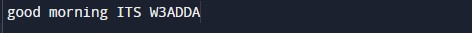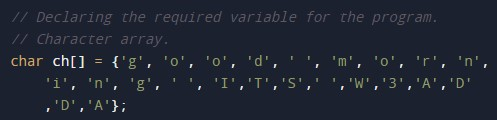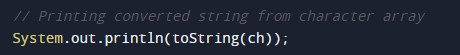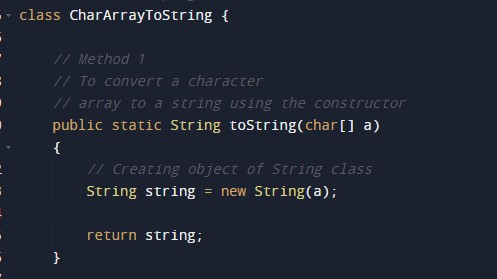# Java Program to convert char Array to String

In this tutorial you will learn about the Java Program to convert char Array to String and its application with practical example.

## Java Program to convert char Array to String

In this tutorial, we will learn to create a Java program that will convert char Array to String using Java programming.

## Prerequisites.

Before starting with this tutorial, we assume that you are the best aware of the following C programming topics:

• Operators in Java Programming.
• Basic Input and Output function in Java Programming.
• Basic Java programming.
• For loop in Java programming.
• Conditional Statements in Java programming.

## Program to convert char Array to String.

In this program, First, we will declare the array in the java program. Then we will define a  function to convert a character array to a string. Then we will return the string to the main function. At last, we will print the string to the user.

With the help of this program, we can convert char Array to String.

## Output:-

character to string output.In the above program, we have first initialized the required variable.• ch[] = it will hold a character array declared.

Passing that character array to the function for conversion.A user-defined function that will convert the string from the Character array.Printing the string by calling the function from the logical code.In this tutorial we have learn about the Java Program to convert char Array to String and its application with practical example. I hope you will like this tutorial.